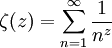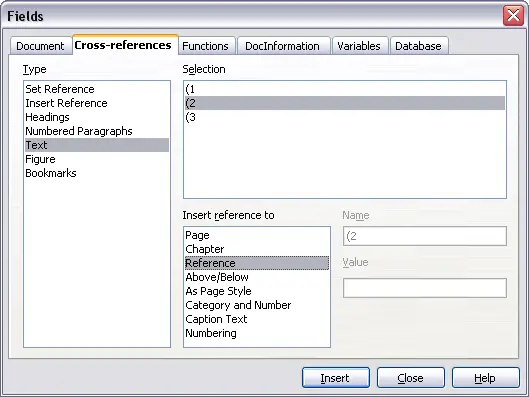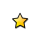On-line GuidesAll GuideseBook StoreiOS / AndroidLinux for BeginnersOffice ProductivityLinux InstallationLinux SecurityLinux UtilitiesLinux VirtualizationLinux KernelSystem/Network AdminProgrammingScripting LanguagesDevelopment ToolsWeb DevelopmentGUI Toolkits/DesktopDatabasesMail SystemsopenSolarisEclipse DocumentationTechotopia.comVirtuatopia.comHow To GuidesVirtualizationGeneral System AdminLinux SecurityLinux FilesystemsWeb ServersGraphics & DesktopPC HardwareWindowsProblem SolutionsPrivacy PolicyOpenOffice 3.x Getting Started Guide
Previous Page Home Next Page

## Numbering equations

Equation numbering is one of OOoMath's best hidden features. The steps are simple, but obscure:

1. Start a new line.
2. Type “fn" and then press F3.

The “fn" is replaced by a numbered formula:

E = mc2 (2)

Now you can double-click on the formula to edit it. For example, here is the Riemann Zeta function:$\zeta (z) = \sum_{n=1}^\infin \frac{1}{n^z}$ (3)

You can reference an equation (“as shown in Equation (2)") with these steps:

1. Insert > Cross-reference..
2. Click on the References tab (Figure 15).
3. Under Type, select Text.
4. Under Selection, pick the equation number.
5. Under Format, choose Reference.
6. Click Insert.

Done! If you later add more equations to the paper before the referenced equation, all the equations will automatically renumber and the cross-references will update.To insert the equation number without parenthesis around it, choose Numbering under Format instead of Reference.

OpenOffice 3.x Getting Started Guide
Previous Page Home Next PagePublished under the terms of the Creative Commons License Design by Interspire